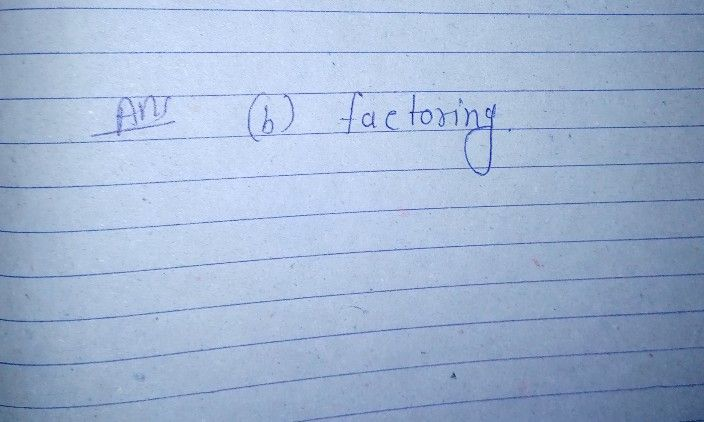Symbol
Problem$2.$ What is the most appropriate method to solve the quadratic equation $x^{2}=47$ a. extracting the square root c. completing the square b. factoring d. using the quadratic formula
7th-9th grade
Other
Search count: 105
SolutionQanda teacher - RASHMIKAif you have any doubt so pls ask me to know